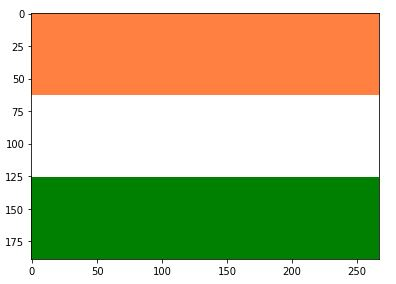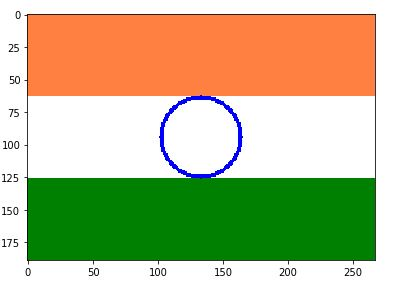# Program to make Indian Flag in Python | Step by Step

Here, We’ll be making Indian Flag in Python using Spyder tool which is a part of Anaconda interface. It is the beginner’s practice problem for python programmers.

Prerequisite: The only prerequisite for this code is little knowledge of basic python programming. You may install anaconda from here if you don’t have any interpreter for python.

Approach: We will be using simple characteristic equations of lines and circles in this code. It is recommended to try on your own before getting to the solution altogether.

Import these 3 packages for the functions used in this code.

```import skimage               # for using image processing functions
import os
from skimage import io       # for using imshow function
```

1. Read an image of at least the expected dimensions of the flag.

```image2 = io.imread('C:\Users\geeksforgeeks\Desktop\index.jpg')
```

2. Let us assume the dimensions of the flag to be 189px high and 267px wide.

```x = 189/2
y = 267/2
r = 189/6
```

3. Start two for loops to iterate to every pixel of the image.

```for i in range(0, 189):
for j in range(0, 267):
```

Inside the inner loop perform 3-(a) and 3-(b).

3-(a). By dividing the image into 3 equal part height-wise color the image in orange, white and green.

```if i in range(0, 189/3):
image2[i][j] = [255, 128, 64]       # Color coding for Orange
elif i in range(189/3, 189*2/3):
image2[i][j] = [255, 255, 255]      # Color coding for White
else:
image2[i][j] = [0, 128, 0]          # Color coding for Green
```io.imshow(image2)

3-(b). Now it’s time to draw the circle at the center for the Ashoka Chakra, using equation of circle.

```if (( pow((x-i), 2) + pow((y-j), 2) ) = pow(r-2, 2)):
image2[i][j] = [0, 0, 255]
```io.imshow(image2)

4. To draw spokes of the Chakra, we need to use equation of lines which stays inside the circle only, here I have drawn 4 x 2 spokes, however all 24 spokes can be drawn by using 12 equations as each line leads to two opposite spokes.

```for i in range(189/3, 189*2/3):
for j in range(267/3, 267*2/3):
if ((i == 189/2) or (j == 267/2) or (i+39 == j or
(i+39+j == 266))) and (( pow((x-i), 2) +
pow((y-j), 2) ) <= pow(r, 2)) :
image2[i][j] = [0, 0, 255]

```

5. It’s time to view our image now so we will use <imshow function.

```io.imshow(image2)
```

And Over Indian Flag in just 5 steps.

The overall code will look something like this…

 `""" ` `@author: geeksforgeeks ` `"""` `# Packages ` `import` `skimage ` `import` `os ` `from` `skimage ``import` `io ` ` `  `# Reading image file ` `image2 ``=` `io.imread(``'C:\Users\KRISHNA\Desktop\index.jpg'``) ` ` `  `# Setting dimensions boundaries ` `x ``=` `189` `/` `2` `y ``=` `267` `/` `2` `r ``=` `189` `/` `6` ` `  `# Iterating to all the pixels in the set boundaries ` `for` `i ``in` `range``(``0``, ``189``): ` `    ``for` `j ``in` `range``(``0``, ``267``): ` `        ``if` `i ``in` `range``(``0``, ``189` `/` `3``): ` `            ``image2[i][j] ``=` `[``255``, ``128``, ``64``]     ``# Orange Color ` `        ``elif` `i ``in` `range``(``189` `/` `3``, ``189` `*` `2` `/` `3``): ` `            ``image2[i][j] ``=` `[``255``, ``255``, ``255``]    ``# White Color ` `        ``else``: ` `            ``image2[i][j] ``=` `[``0``, ``128``, ``0``]        ``# Green Color ` `             `  `            ``# Equation for 2px thick ring ` `        ``if` `(( ``pow``((x``-``i), ``2``) ``+` `pow``((y``-``j), ``2``) ) <``=` `pow``(r ``+` `1``, ``2``)) ``and``(( ``pow``((x``-``i), ``2``) ``+` `pow``((y``-``j), ``2``) ) >``=` `pow``(r``-``2``, ``2``)): ` `            ``image2[i][j] ``=` `[``0``, ``0``, ``255``]        ``# Blue Color ` `             `  `# Iterating within the ring  ` `for` `i ``in` `range``(``189` `/` `3``, ``189` `*` `2` `/` `3``): ` `    ``for` `j ``in` `range``(``267` `/` `3``, ``267` `*` `2` `/` `3``): ` `         `  `        ``# Equation to draw 4 straight lines ` `        ``if` `((i ``=``=` `189` `/` `2``) ``or` `(j ``=``=` `267` `/` `2``) ``or` `(i ``+` `39` `=``=` `j ``or` `(i ``+` `39` `+` `j ``=``=` `266``))) ``and` `(( ``pow``((x``-``i), ``2``) ``+` `pow``((y``-``j), ``2``) ) <``=` `pow``(r, ``2``)) : ` `            ``image2[i][j] ``=` `[``0``, ``0``, ``255``]        ``# Blue Color ` ` `  `io.imshow(image2)                           ``# Output `

Output:Output

My Personal Notes arrow_drop_upCheck out this Author's contributed articles.

If you like GeeksforGeeks and would like to contribute, you can also write an article using contribute.geeksforgeeks.org or mail your article to contribute@geeksforgeeks.org. See your article appearing on the GeeksforGeeks main page and help other Geeks.

Please Improve this article if you find anything incorrect by clicking on the "Improve Article" button below.

Article Tags :

2

Please write to us at contribute@geeksforgeeks.org to report any issue with the above content.